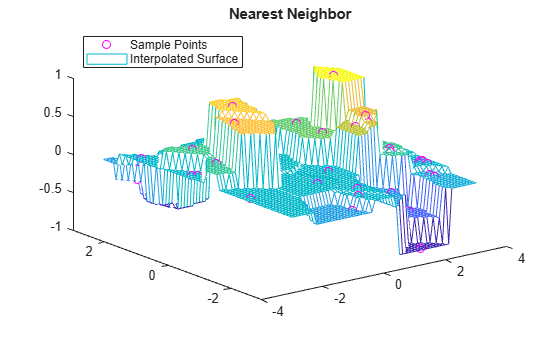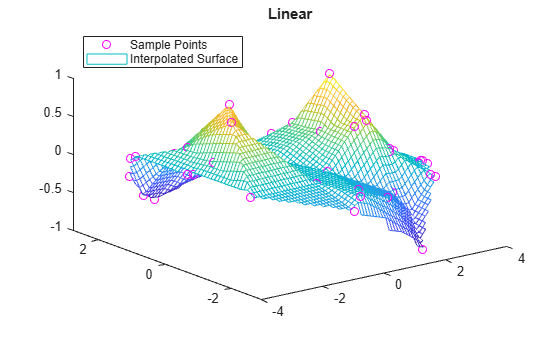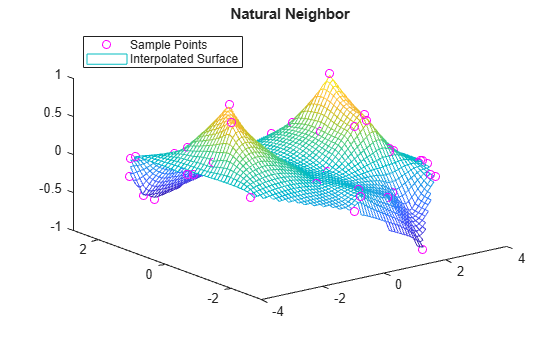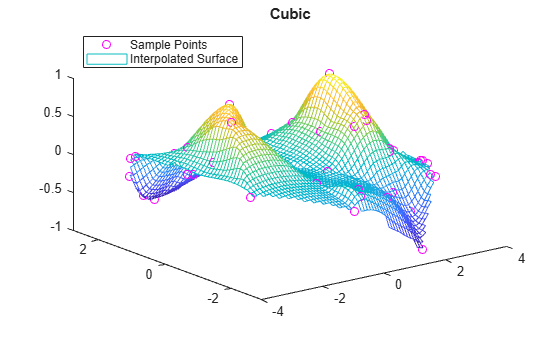# griddata

## 语法

``vq = griddata(x,y,v,xq,yq)``
``vq = griddata(x,y,z,v,xq,yq,zq)``
``vq = griddata(___,method)``
``[Xq,Yq,vq] = griddata(x,y,v,xq,yq)``
``[Xq,Yq,vq] = griddata(x,y,v,xq,yq,method)``

## 说明

``vq = griddata(x,y,v,xq,yq)` 使 v = f(x,y) 形式的曲面与向量 `(x,y,v)` 中的散点数据拟合。`griddata` 函数在 `(xq,yq)` 指定的查询点对曲面进行插值并返回插入的值 `vq`。曲面始终穿过 `x` 和 `y` 定义的数据点。`

``vq = griddata(x,y,z,v,xq,yq,zq)` 拟合 v = f(x,y,z) 形式的超曲面。`
``vq = griddata(___,method)` 使用上述语法中的任何输入参数指定计算 `vq` 所用的插值方法。`method` 可以是 `'linear'`、`'nearest'`、`'natural'`、`'cubic'` 或 `'v4'`。默认方法为 `'linear'`。`
``[Xq,Yq,vq] = griddata(x,y,v,xq,yq)` 和 `[Xq,Yq,vq] = griddata(x,y,v,xq,yq,method)` 还返回 `Xq` 和 `Yq`，其中包含查询点的网格坐标。`

## 示例

```rng('default') xy = -2.5 + 5*rand([200 2]); x = xy(:,1); y = xy(:,2); v = x.*exp(-x.^2-y.^2);```

`x``y``v` 是包含分散（非均匀）样本点和数据的向量。

```[xq,yq] = meshgrid(-2:.2:2, -2:.2:2); vq = griddata(x,y,v,xq,yq);```

```mesh(xq,yq,vq) hold on plot3(x,y,v,'o') xlim([-2.7 2.7]) ylim([-2.7 2.7])``````x = 2*rand(2500,1) - 1; y = 2*rand(2500,1) - 1; z = 2*rand(2500,1) - 1; v = x.^2 + y.^3 - z.^4;```

```d = -1:0.05:1; [xq,yq,zq] = meshgrid(d,d,0);```

```vq = griddata(x,y,z,v,xq,yq,zq); plot3(x,y,v,'ro') hold on surf(xq,yq,vq)``````x = -3 + 6*rand(50,1); y = -3 + 6*rand(50,1); v = sin(x).^4 .* cos(y);```

`[xq,yq] = meshgrid(-3:0.1:3);`

```z1 = griddata(x,y,v,xq,yq,'nearest'); plot3(x,y,v,'mo') hold on mesh(xq,yq,z1) title('Nearest Neighbor') legend('Sample Points','Interpolated Surface','Location','NorthWest')``````z2 = griddata(x,y,v,xq,yq,'linear'); figure plot3(x,y,v,'mo') hold on mesh(xq,yq,z2) title('Linear') legend('Sample Points','Interpolated Surface','Location','NorthWest')``````z3 = griddata(x,y,v,xq,yq,'natural'); figure plot3(x,y,v,'mo') hold on mesh(xq,yq,z3) title('Natural Neighbor') legend('Sample Points','Interpolated Surface','Location','NorthWest')``````z4 = griddata(x,y,v,xq,yq,'cubic'); figure plot3(x,y,v,'mo') hold on mesh(xq,yq,z4) title('Cubic') legend('Sample Points','Interpolated Surface','Location','NorthWest')``````figure plot3(x,y,v,'mo') hold on mesh(xq,yq,sin(xq).^4 .* cos(yq)) title('Exact Solution') legend('Sample Points','Exact Surface','Location','NorthWest')```## 输入参数

`'linear'`基于三角剖分的线性插值（默认），支持二维和三维插值。C0
`'nearest'`基于三角剖分的最近邻点插值，支持二维和三维插值。不连续
`'natural'`基于三角剖分的自然邻点插值，支持二维和三维插值。该方法在线性与立方之间达到有效的平衡。C1，样本点处除外
`'cubic'`基于三角剖分的三次插值，仅支持二维插值。C2
`'v4'`

C2

## 输出参数

• 对于二维插值（其中 `xq``yq` 指定查询点的 `m`×`n` 网格），`vq``m`×`n` 数组。

• 对于三维插值（其中 `xq``yq``zq` 指定查询点的 `m`×`n`×`p` 网格），`vq``m`×`n`×`p` 数组。

• 如果 `xq``yq`（对于三维插值还有 `zq`）是指定散点的向量，则 `vq` 是长度相同的向量。

• 如果将 `xq` 指定为行向量，将 `yq` 指定为列向量，则 `griddata` 使用这些网格向量通过 `[Xq,Yq] = meshgrid(xq,yq)` 来构成完整网格。在这种情况下，`Xq``Yq` 输出以包含查询点的完整网格坐标的矩阵形式返回。

• 如果 `xq``yq` 均为行向量或均为列向量，则 `Xq = xq``Yq = yq`